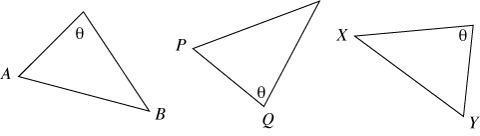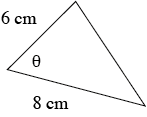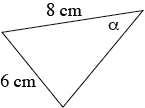Home > Geometric reasoning > Big ideas > Congruence > Congruent triangles

# Congruent triangles

By constructing triangles which satisfy three given conditions, students realise that there can be more than one triangle for a given angle size and two given side lengths.

There are four commonly used congruence tests.

1. Side Side Side (SSS)
The three sides of one triangle are respectively equal to the three sides of the other triangle.
2. Side Angle Side (SAS)
Two sides and the included angle of one triangle are respectively equal to two sides and the included angle of the other triangle.
3. Angle Angle Side (AAS)
Two angles and one side of one triangle are respectively equal to two angles and the matching side of the other triangle.
4. Right angle Hypotenuse Side (RHS)
The hypotenuse and one side of one right‐angled triangle are respectively equal to the hypotenuse and one side of the other right‐angled triangle.

Within the tests, reference is made to matching sides and included angles.

• Matching sides are opposite equal angles. Which sides match?AB and XY are matching sides as they are both opposite $$\theta$$. PQ is not a matching side.

• Included angles are formed by the equal sides. Which angle is included?$$\theta$$ is the included angle.$$\alpha$$ is not the included angle.